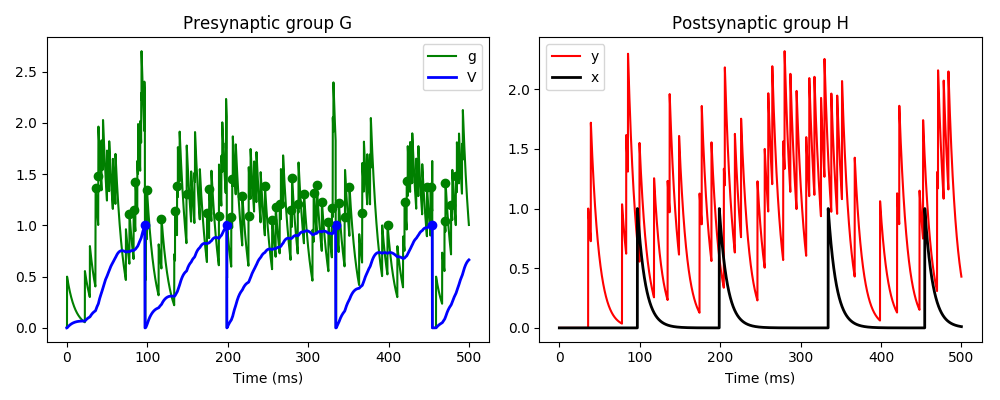# Example: custom_events¶

Note

You can launch an interactive, editable version of this example without installing any local files using the Binder service (although note that at some times this may be slow or fail to open):Example demonstrating the use of custom events.

Here we have three neurons, the first is Poisson spiking and connects to neuron G, which in turn connects to neuron H. Neuron G has two variables v and g, and the incoming Poisson spikes cause an instantaneous increase in variable g. g decays rapidly, and in turn causes a slow increase in v. If v crosses a threshold, it causes a standard spike and reset. If g crosses a threshold, it causes a custom event `gspike`, and if it returns below that threshold it causes a custom event `end_gspike`. The standard spike event when v crosses a threshold causes an instantaneous increase in variable x in neuron H (which happens through the standard `pre` pathway in the synapses), and the gspike event causes an increase in variable y (which happens through the custom pathway `gpath`).

```from brian2 import *
# Input Poisson spikes
inp = PoissonGroup(1, rates=250*Hz)
# First group G
eqs_G = '''
dv/dt = (g-v)/(50*ms) : 1
dg/dt = -g/(10*ms) : 1
allow_gspike : boolean
'''
G = NeuronGroup(1, eqs_G, threshold='v>1',
reset='v = 0; g = 0; allow_gspike = True;',
events={'gspike': 'g>1 and allow_gspike',
'end_gspike': 'g<1 and not allow_gspike'})
G.run_on_event('gspike', 'allow_gspike = False')
G.run_on_event('end_gspike', 'allow_gspike = True')
# Second group H
eqs_H = '''
dx/dt = -x/(10*ms) : 1
dy/dt = -y/(10*ms) : 1
'''
H = NeuronGroup(1, eqs_H)
# Synapses from input Poisson group to G
Sin = Synapses(inp, G, on_pre='g += 0.5')
Sin.connect()
# Synapses from G to H
S = Synapses(G, H,
on_pre={'pre': 'x += 1',
'gpath': 'y += 1'},
on_event={'pre': 'spike',
'gpath': 'gspike'})
S.connect()
# Monitors
Mstate = StateMonitor(G, ('v', 'g'), record=True)
Mgspike = EventMonitor(G, 'gspike', 'g')
Mspike = SpikeMonitor(G, 'v')
MHstate = StateMonitor(H, ('x', 'y'), record=True)
# Initialise and run
G.allow_gspike = True
run(500*ms)
# Plot
figure(figsize=(10, 4))
subplot(121)
plot(Mstate.t/ms, Mstate.g, '-g', label='g')
plot(Mstate.t/ms, Mstate.v, '-b', lw=2, label='V')
plot(Mspike.t/ms, Mspike.v, 'ob', label='_nolegend_')
plot(Mgspike.t/ms, Mgspike.g, 'og', label='_nolegend_')
xlabel('Time (ms)')
title('Presynaptic group G')
legend(loc='best')
subplot(122)
plot(MHstate.t/ms, MHstate.y, '-r', label='y')
plot(MHstate.t/ms, MHstate.x, '-k', lw=2, label='x')
xlabel('Time (ms)')
title('Postsynaptic group H')
legend(loc='best')
tight_layout()
show()
```